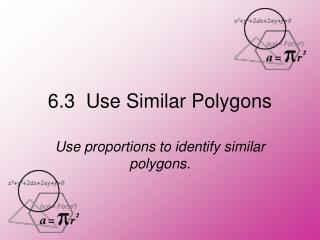DownloadDownload Presentation6.3 Use Similar Polygons

# 6.3 Use Similar Polygons

Download Presentation## 6.3 Use Similar Polygons

- - - - - - - - - - - - - - - - - - - - - - - - - - - E N D - - - - - - - - - - - - - - - - - - - - - - - - - - -
##### Presentation Transcript

1. 6.3 Use Similar Polygons Use proportions to identify similar polygons.

2. Vocabulary • Two polygons are similar polygons if corresponding angles are congruent and corresponding side lengths are proportional.

3. In the diagram, ∆RST ~ ∆XYZ a. List all pairs of congruent angles. b. Check that the ratios of corresponding side lengths are equal. c. Write the ratios of the corresponding side lengths in a statement of proportionality. EXAMPLE 1 Use similarity statements

4. a. 5 ST 30 25 TR 5 RS 5 20 b. ; ; = = = = = = 3 15 3 ZX 3 18 XY 12 YZ ~ ~ ~ R X, T Z and Y S = = = . TR ST RS = = YZ ZX XY EXAMPLE 1 Use similarity statements SOLUTION c. Because the ratios in part (b) are equal,

5. 1. Given ∆ JKL ~ ∆ PQR, list all pairs of congruent angles. Write the ratios of the corresponding side lengths in a statement of proportionality. L R ~ ~ ~ The congruent angles are J P, L R and Q K = = = Q K J P JK LJ KL = = RP PQ QR The ratios of the corresponding side lengths are. for Example 1 GUIDED PRACTICE SOLUTION

6. Vocabulary • If two polygons are similar, then the ratio of the lengths or two corresponding sides is the called the scale factor.

7. Determine whether the polygons are similar. If they are, write a similarity statement and find the scale factor of ZYXWto FGHJ. EXAMPLE 2 Find the scale factor

8. ALGEBRA In the diagram, ∆DEF ~ ∆MNP.Find the value of x. EXAMPLE 3 Use similar polygons x = 15

9. for Examples 2 and 3 GUIDED PRACTICE In the diagram, ABCD ~ QRST. 2. What is the scale factor of QRSTto ABCD ?

10. In the diagram, ABCD ~ QRST. x 8 = for Examples 2 and 3 GUIDED PRACTICE 3. Find the value of x.

11. Theorem 6.1: Perimeters of Similar Polygons • If two polygons are similar, then the ratio of their perimeters is equal to the ratios of their corresponding side lengths.

12. b. Swimming Find the perimeter of an Olympic pool and the new pool. A town is building a new swimming pool. An Olympic pool is rectangular with length 50 meters and width 25 meters. The new pool will be similar in shape, but only 40 meters long. a. Find the scale factor of the new pool to an Olympic pool. EXAMPLE 4 Find perimeters of similar figures

13. In the diagram, ABCDE ~ FGHJK. ANSWER 3 The scale factor is the ratio of the length is 15 2 10 = for Example 4 GUIDED PRACTICE 4. Find the scale factor of FGHJKto ABCDE.

14. In the diagram, ABCDE ~ FGHJK. for Example 4 GUIDED PRACTICE 5. Find the value of x. x = 12

15. In the diagram, ABCDE ~ FGHJK. for Example 4 GUIDED PRACTICE 6. Find the perimeter of ABCDE. The perimeter of ABCDE is 46 units

16. Similarity and Congruence • Notice that any two congruent figures are also similar. Their scale factor is 1:1. In triangles ABC and DEF, the scale factor is 5:5 = 1.

17. Corresponding Lengths • You know that perimeters of similar polygons are in the same ratio as a corresponding side lengths. You can extend this concept to other segments in polygons.

18. Key Concept: Corresponding Lengths in Similar Polygons • If two polygons are similar, then the ratio of any two corresponding lengths in the polygons is equal to the scale factor of the similar polygons.

19. In the diagram, ∆TPR~∆XPZ. Find the length of the altitude PS. The length of the altitude PSis 15. EXAMPLE 5 Use a scale factor ANSWER

20. 7. In the diagram, ∆JKL ~ ∆ EFG. Find the length of the median KM. KM = 42 for Example 5 GUIDED PRACTICE In the diagram, ABCDE ~ FGHJK.

21.  Homework  Page 376/1,2,4-10,14-18,23-26,29-32